# TI-30 eco RS Scientific Calculator

Basic solar-powered scientific calculator with one-line display and general math and science functionality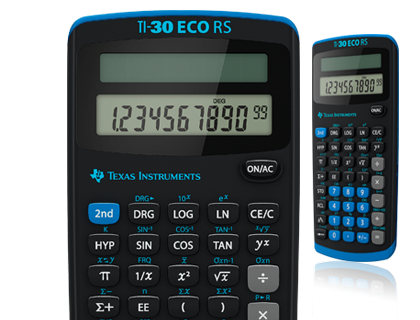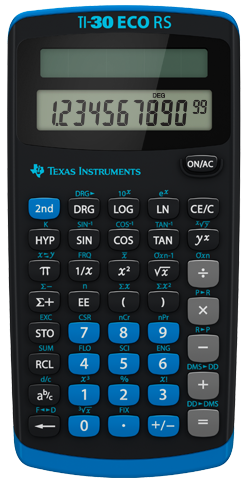#### Key Features

• Made out of 100% recycled plastics
• One-line, 10-digit display
• Fraction features
• One-variable statistics
• Conversions
• Basic scientific and trigonometric functions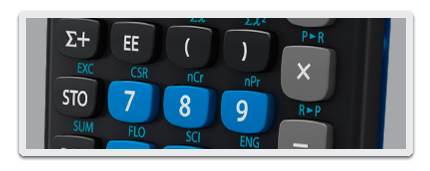## Perform operations with fractions and mixed numbers

Adds, subtracts, multiplies and divides fractions entered in traditional numerator/denominator format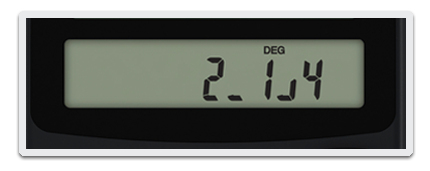## Calculate basic conversions

• Polar/rectangular
• DMS/degrees
• Fraction/decimal## Specifications#### Built-in functionality

• Fraction/decimal conversions
• Change improper fractions to mixed numbers
• Automatic simplification of fractions
• Negation key
• One constant
• Combinations and permutations
• Trigonometry
• Hyperbolics
• Logs and antilogs
• Convert angles from degrees to radians to grads
• %, x², x3, ¹/x, xˆy, π, x!
• Fixed decimal capability
• One-variable statistics
• AOS: (Algebraic Operating System)
• Three memory variables
• Scientific and engineering notation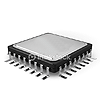#### Hardware

• Hard plastic, color-coded keys
• One-line, 10-digit display
• Solar power
• Impact-resistant cover